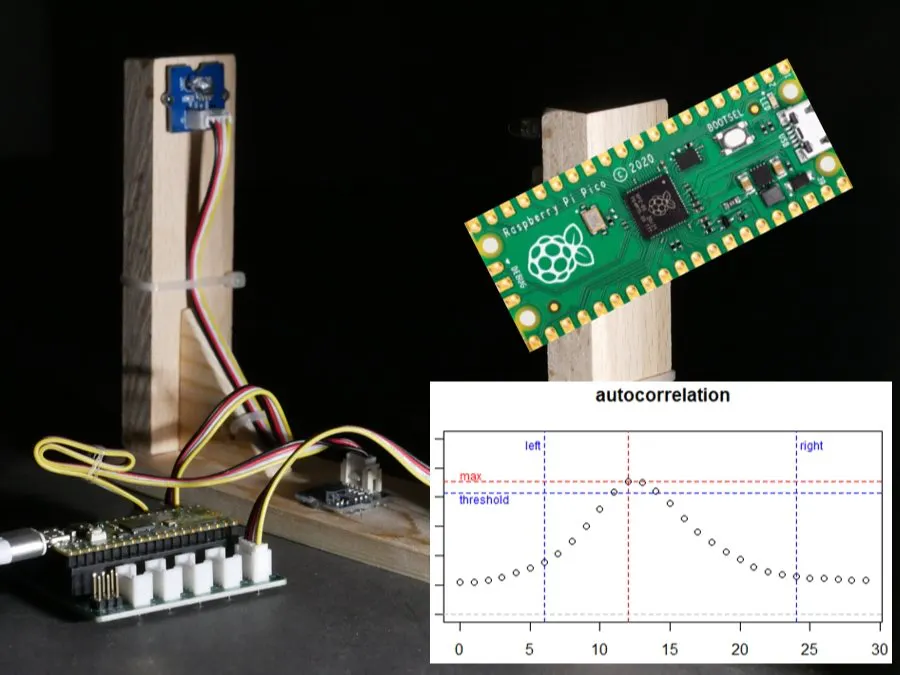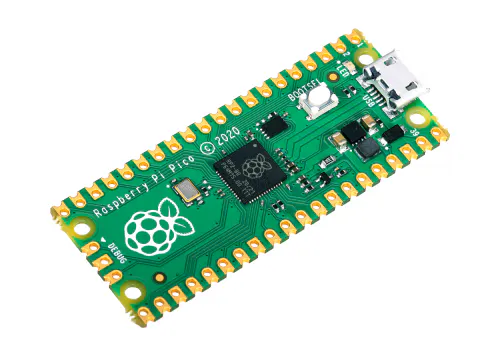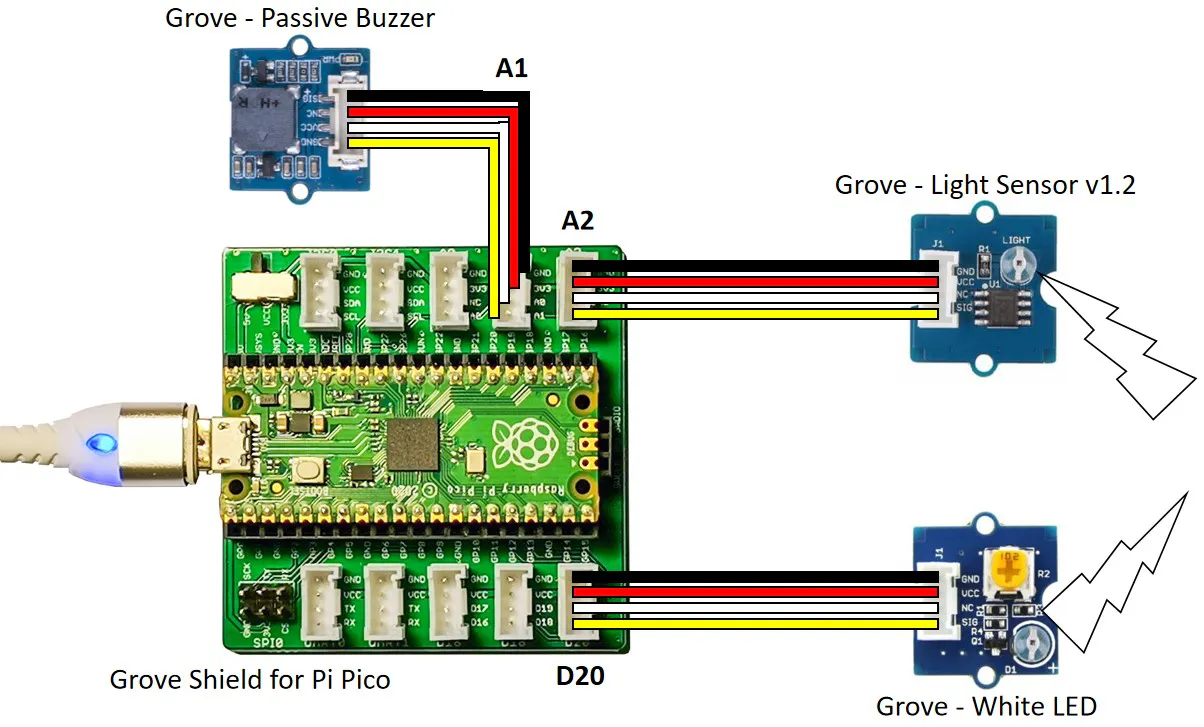# Over-engineered dual core light barrier

If the Raspberry Pi Pico already has two cores, why not use both for a light barrier with Barker-coded pulse sequences and autocorrelation?

BeginnerFull instructions provided3 hours3,985## Things used in this project

### Hardware components

 Seeed Studio Grove Starter Kit for Raspberry Pi Pico
×1Raspberry Pi Pico
×1

### Software apps and online services

 ThonnyMicroPython

## Schematics

### Schematics of LED light barrier## Code

### micropython code for light barrier

MicroPython
the code to run the light barrier
```###################################################
# Over-engineered dual core light barrier
# If the Raspberry Pi Pico already has two cores,
# why not use both for a light barrier with Barker-
# coded pulse sequences and autocorrelation?
#
# Hague Nusseck @ electricidea
# v1.0 | 27.December.2022
# https://github.com/electricidea/pico_light_barrier
#
#
# Check the complete project at Hackster.io:
# https://www.hackster.io/hague/over-engineered-dual-core-light-barrier-c523a9
#
# Distributed as-is; no warranty is given.
####################################################

import utime

led = Pin(20, Pin.OUT)                     # led connect to D20
light_sensor = ADC(2)                      # light sensor connected to A2
pwm = PWM(Pin(27))                         # buzzer connected to A1
pwm.freq(10000)

# Length and frequency of the sampling of the light sensor
n_samples = 75                             # number of samples captured from light sensor
sample_freq = 5000                         # sample frequency in Hz (5kHz are recommended)
dt_samples = (1 / sample_freq) * 1000000   # resulting time between two samples in microseconds

Barker_Code = [ 1.0,  1.0,  1.0,  0.0,  0.0,  0.0,  1.0,  0.0,  0.0,  1.0,  0.0] # length 11

# calculate the length of one "Barker Pulse" based on the length
# of the sampling window.
# The length is calculated by the number of pulses of the Barker Code
# plus 2 additional pulses (larger sampling window, shorter LED sequence)
pulse_length = n_samples // (len(Barker_Code)+2)
# generate the Barker Code as an array with the timing of
# the sampling frequency for the autocorrelation
n_Barker_Code_samples = len(Barker_Code)*pulse_length
Barker_Code_samples = [0.0] * n_Barker_Code_samples
for n in range(len(Barker_Code)):
for i in range(pulse_length):
Barker_Code_samples[n*pulse_length+i] = Barker_Code[n]

# increase the sampling windows by some "Barker Pulses" to create
# enough room for shifting for the autocorrelation
n_samples = n_samples + round(len(Barker_Code)/5)*pulse_length
# data array to store the measured sample data
data = [0.0] * n_samples
# array to store the sums of the auto correlation
n_autocorr = n_samples - n_Barker_Code_samples
autocorr = [0.0] * n_autocorr
autocorr_valid_window = [round(n_autocorr * 0.2), n_autocorr-round(n_autocorr * 0.2)]

# This wait function is not waiting for a fix number of ticks
# it is waiting until the actual tick-value (in microseconds)
# reaches the sum of the start-value and the add-value.
# With this method, a more precise pulse generation and
# sampling timing can be reached without adding up errors
# The functions utime.ticks_add() and utime.ticks_diff() are
# used to prevent value overflow problems with tick variables.
while utime.ticks_diff(wait_time, utime.ticks_us()) >= 0:
pass

# The LED should be pulsed in its own task on the second core
# so we need a own function to switch the LED on and off to
# generate the light pulse pattern
led.value(False)
led_start_ticks_us = utime.ticks_us()
# wait two pulse lenghts before start sequence
wait_until_ticks(led_start_ticks_us, 2*pulse_length*dt_samples)
for n, led_on_off in enumerate(Barker_Code):
if led_on_off > 0:
led.value(True)
else:
led.value(False)
wait_until_ticks(led_start_ticks_us, (n+3)*pulse_length*dt_samples)
led.value(False)

# simple buzzer-beep function
def beep(freq, duration=0.125):
pwm.freq(freq)
pwm.duty_u16(10000)
utime.sleep(0.125)
pwm.duty_u16(0)

# function to start the pulse generation an the measurement
# of the data from the light sensor
# the measured values are scaled from 0..0xFFFF to 0..1
# return value: minimum of all measured values
def measure():
# the minimum value is used to compensate the offset
# during the autocorrelation calculation
light_val_min = 0xFFFF
# start the led pulse generation on the second core
start_ticks_us = utime.ticks_us()
for n in range(n_samples):
data[n] = light_val
if light_val < light_val_min:
light_val_min = light_val
wait_until_ticks(start_ticks_us, (n+1)*dt_samples)
return (light_val_min)

# function to calculate the autocorrelation between the pulse signal
# and the measured light sensor data
# the minimum value is used to compensate the offset of the measurement
def autocorrelation(_min_val):
autocorr_threshold = 0.75
autocorr_max = -1000
autocorr_max_pos = 0
detection_state = 1
signal_detected = False
for shift in range(n_autocorr):
autocorr[shift] = 0
for pos in range(n_Barker_Code_samples):
autocorr[shift] += Barker_Code_samples[pos] * (data[pos+shift]-_min_val)
# detection state 1: looking for maximum
if detection_state == 1:
if autocorr[shift] > autocorr_max:
autocorr_max = autocorr[shift]
autocorr_max_pos = shift
# When a falling curve is detected and max is within the valid range and position
if (autocorr[shift] < autocorr_max) and ((autocorr_max - autocorr) > autocorr_threshold) and (autocorr_max_pos >= autocorr_valid_window):
if autocorr_max_pos > autocorr_valid_window:
detection_state = 3
else:
detection_state = 2
# detection state 2: check for valid falling curve
if detection_state == 2:
if (autocorr[shift] < (autocorr_max - autocorr_threshold)):
signal_detected = True
detection_state = 3
#print(light_val_mean, autocorr_max, autocorr_max_pos)
return (signal_detected)

# main loop
while True:
if not autocorrelation(measure()):
beep(800)
```

### github repository

all code files and R analyzing script

## Credits

### Hans-Günther Nusseck

16 projects • 36 followers
Just a guy who can't pass by any hardware without wondering how it works. Managing robot based industrial automation projects for living.